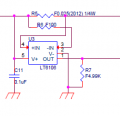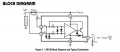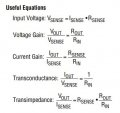Chandler Timm Doloriel

Joined Apr 5, 2018
54
I couldn't convert the ADC reading to its respective analog current value.
I connected a microcontroller in the Vout to read the current, it will give an 8bit HEX value.

(8-bit @ 3.3V) and LTC6106 for current sensing. Here's my schematic.The input voltage is 3.3V.
My expected value is 172mA

Here's my calculation:

3.3 V / (2^8 - 1) = 12.94 mV.
Sensitivity = V_out / I_sense = R_sense * R_out/ R_in, = 0.025 Ω * (4999 Ω) / 100 Ω = 1.25 V/A
I_out = 12.94 mV * 22 / 1.25 V/A = 227.744 mA

What's my mistake here? Why I did not get 172mA?

Here are useful info from the datasheet of LTC6106ericgibbs

Joined Jan 29, 2010
14,693
hi CTD,
Your calculations are correct, I will look over the LT6106 d/s.
E

Update:

How is R5, the Shunt Mounted/Fixed, additional resistance in the mounting will give you a higher current reading.

Do you have a photo image to post.?

Chandler Timm Doloriel

Joined Apr 5, 2018
54
hi CTD,
Your calculations are correct, I will look over the LT6106 d/s.
E

Update:
How is R5, the Shunt Mounted/Fixed, additional resistance in the mounting will give you a higher current reading.

Do you have a photo image to post.?
Thanks! I can't share the picture of my board/set-up, unfortunately.

I was also thinking of improving the Rsense, the Vref, and 16bit instead of 8bit. Will it solve my problem?

ericgibbs

Joined Jan 29, 2010
14,693
hi,
As I cannot see your set up, it is difficult to suggest a fix.
Try this,
Reverse calculate exactly what the value of R5 resistor would have to be to give you a 227mA reading